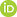Full Text:<2015>

Summary:<1483>

CLC number: TP13

On-line Access: 2016-06-06

Revision Accepted: 2016-04-28

Crosschecked: 2016-05-23

Cited: 0

Clicked: 5103

Citations:  Bibtex RefMan EndNote GB/T7714ORCID:

Bin-bin Lei

http://orcid.org/0000-0003-3612-5625

### -   Go to

Article info.
 Frontiers of Information Technology & Electronic Engineering  2016 Vol.17 No.6 P.587-602 http://doi.org/10.1631/FITEE.1601019Derivation and analysis on the analytical structure of interval type-2 fuzzy controller with two nonlinear fuzzy sets for each input variable

 Author(s):  Bin-bin Lei, Xue-chao Duan, Hong Bao, Qian Xu Affiliation(s):  MOE Key Laboratory of Electronic Equipment Structure Design, Xidian University, Xi’an 710071, China; more Corresponding email(s):   18717368076@163.com Key Words:  Interval type-2 fuzzy controller, Analytical structure, Karnik-Mendel type reducer Share this article to： More <<< Previous Article|

Bin-bin Lei, Xue-chao Duan, Hong Bao, Qian Xu. Derivation and analysis on the analytical structure of interval type-2 fuzzy controller with two nonlinear fuzzy sets for each input variable[J]. Frontiers of Information Technology & Electronic Engineering, 2016, 17(6): 587-602.

@article{title="Derivation and analysis on the analytical structure of interval type-2 fuzzy controller with two nonlinear fuzzy sets for each input variable",
author="Bin-bin Lei, Xue-chao Duan, Hong Bao, Qian Xu",
journal="Frontiers of Information Technology & Electronic Engineering",
volume="17",
number="6",
pages="587-602",
year="2016",
publisher="Zhejiang University Press & Springer",
doi="10.1631/FITEE.1601019"
}

%0 Journal Article
%T Derivation and analysis on the analytical structure of interval type-2 fuzzy controller with two nonlinear fuzzy sets for each input variable
%A Bin-bin Lei
%A Xue-chao Duan
%A Hong Bao
%A Qian Xu
%J Frontiers of Information Technology & Electronic Engineering
%V 17
%N 6
%P 587-602
%@ 2095-9184
%D 2016
%I Zhejiang University Press & Springer
%DOI 10.1631/FITEE.1601019

TY - JOUR
T1 - Derivation and analysis on the analytical structure of interval type-2 fuzzy controller with two nonlinear fuzzy sets for each input variable
A1 - Bin-bin Lei
A1 - Xue-chao Duan
A1 - Hong Bao
A1 - Qian Xu
J0 - Frontiers of Information Technology & Electronic Engineering
VL - 17
IS - 6
SP - 587
EP - 602
%@ 2095-9184
Y1 - 2016
PB - Zhejiang University Press & Springer
ER -
DOI - 10.1631/FITEE.1601019

Abstract:
Type-2 fuzzy controllers have been mostly viewed as black-box function generators. Revealing the analytical structure of any type-2 fuzzy controller is important as it will deepen our understanding of how and why a type-2 fuzzy controller functions and lay a foundation for more rigorous system analysis and design. In this study, we derive and analyze the analytical structure of an interval type-2 fuzzy controller that uses the following identical elements: two nonlinear interval type-2 input fuzzy sets for each variable, four interval type-2 singleton output fuzzy sets, a Zadeh AND operator, and the karnik-Mendel type reducer. Through dividing the input space of the interval type-2 fuzzy controller into 15 partitions, the input-output relationship for each local region is derived. Our derivation shows explicitly that the controller is approximately equivalent to a nonlinear proportional integral or proportional differential controller with variable gains. Furthermore, by comparing with the analytical structure of its type-1 counterpart, potential advantages of the interval type-2 fuzzy controller are analyzed. Finally, the reliability of the analysis results and the effectiveness of the interval type-2 fuzzy controller are verified by a simulation and an experiment.

### Reference

Du, X.Y., Ying, H., 2007. Control performance comparison between a type-2 fuzzy controller and a comparable conventional Mamdani fuzzy controller. Annual Meeting of the North American Fuzzy Information Processing Society, p.100-105.Du, X.Y., Ying, H., 2010. Derivation and analysis of the analytical structures of the interval type-2 fuzzy-PI and PD controllers. IEEE Trans. Fuzzy Syst., 18(4):802-814.Hagras, H., 2007. Type-2 FLCs: a new generation of fuzzy controllers. IEEE Comput. Intell. Mag., 2(1):30-43.Haj-Ali, A., Ying, H., 2004. Structural analysis of fuzzy controllers with nonlinear input fuzzy sets in relation to nonlinear PID control with variable gains. Automatica, 40(9):1551-1559.Li, Q.C., Shen, D.Y., 2009. Brand-new PID fuzzy controller (fuzzy PI+fuzzy PD). Contr. Dec., 24(7):1037-1042 (in Chinese).Lin, F.J., 2015. Type-2 fuzzy logic control. IEEE Syst. Man Cybern. Mag., 1(1):47-48.Mendel, J.M., 2007. Type-2 fuzzy sets and systems: an overview. IEEE Comput. Intell. Mag., 2(1):20-29.Nie, M.W., Tan, W.W., 2012. Analytical structure and characteristics of symmetric Karnik-Mendel type-reduced interval type-2 fuzzy PI and PD controllers. IEEE Trans. Fuzzy Syst., 20(3):416-430.Wu, D.R., Tan, W.W., 2007. A simplified type-2 fuzzy controller for real-time control. ISA Trans., 45(4):503-516.Ying, H., Siler, W., Buckley, J.J., 1990. Fuzzy control theory: a nonlinear case. Automatica, 26(3):513-520.Zhou, H.B., Ying, H., 2012. A technique for deriving analytical structure of a general class of interval type-2 TS fuzzy controllers. Annual Meeting of the North American Fuzzy Information Processing Society, p.1-6.Zhou, H.B., Ying, H., 2013. A method for deriving the analytical structure of a broad class of typical interval type-2 Mamdani fuzzy controllers. IEEE Trans. Fuzzy Syst., 21(3):447-458.Zhou, H.B., Ying, H., 2014. A method for deriving the analytical structure of the TS fuzzy controllers with two linear interval type-2 fuzzy sets for each input variable. IEEE Int. Conf. on Fuzzy Systems, p.612-618.Zhou, H.B., Ying, H., Duan, J.A., 2009. Adaptive control using interval type-2 fuzzy logic. IEEE Int. Conf. on Fuzzy Systems, p.836-841.